Se muestran los artículos pertenecientes a Octubre de 2017.

## 7th GRADE SCIENCE : Article 18 : Reviewing Newton's laws and third Newton's law.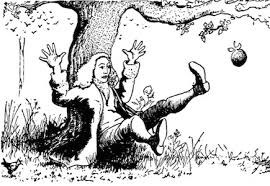THIRD NEWTONS LAW AND REVIEWING.

* Activity 1.24   :   Reviewing Newtons laws.                                                ( 10/9/17   Monday )

1.24.a    Reviewing Newtons laws TEST.           ( 10 points ).

1.24.b    Newtons first laws Word search.          ( 5 points ).

* Activity 1.25   :   Reviewing Newtons laws vocabulary.                         ( 10/10/17   Tuesday )

1.25.a    Reviewing Newtons laws 2.                  ( 10 points ).

1.25.b    Reviewing Newtonss laws 3.                 ( 5 points ).

1.25.c    Reviewing Newtonss laws  vocabulary 4.   ( 5 points ).

* Activity 1.26   :   Third Newtons law.                                                ( 10/11/17   Wednesday )

1.26.a   Look the following webpage up and read about the  Third Newtons law
When you finish Reading it, answer the questions below in a wordfile :
( 10 points ).

* Questions and activities :

-  Who wrote the Third Newtons law?.
-  What does the Third Newtons law say?.
-  What does a rocket have to do with the third Newtons law?.
-  When you push an object, it pushes back.  Is this right?  why?.
-  Remember the reaction force car we made in class a weeks ago.
Explain how the third Newtons law is involved when the car works.
( You can use or make a draw to explain it).
-  Look for an exemple and a picture in which you explain that the third
Newtons law is involved. ( springboard jump ).
-  If you stay just in front of a Wall and you push it hard, what does it
happen?.   Explain it using the third Newtons law.

-  Check the following web out and look at the swimmers picture.
Explain what is happening at the picture using the third Newtons law.

-  Read the following webpage now and explain using your own words
why is so important to consider the third Newtons law in order to build
a rocket. Explain also how the rockets engine Works.

1.26.b   Do the following activity and explain the aftermath after having read the links
above.

- Look at this picture.  Explain what is going to happen and use the third
Newtons law to explain it.                    ( 5 points ).

* Activity 1.27   :   Summarising Newtons laws.                                     ( 10/12/17   Thursday )

1.27.a    Check and read the three Newtons laws at the following web.
Then write a nice outline or summary about them and send it to me by email.

( 10  points ).

1.27.b    Newtons  laws drawing for using in the poster.     ( 10 points ).
Use the given drawings above to show the three Newtons laws in your poster.
At the pink paper given make an outline up showing the 3 Newtons laws
using the drawings given.  You can see an EXAMPLE here about how to do it.

1.27.c   Read the Newtons biography and make a nice summary USING YOUR OWN
WORDS AND TAKING THE INFORMATION YOU CONSIDER MOST IMPORTANT.

( 10  points ).

* Activity 1.28   :   Balanced forces.                                                            ( 10/13/17   Friday )

1.28.a    Copy these paragraphes at the paper given to you today :

1.   " Balanced forces are a consequence of the third Newtons law or also called
action-reaction law of motion. This law is used to explain how forces act when
an objecte is in balance or EQUILIBRIUM and then the object remains in its place
due to the action of several forces acting upon it. Like a lamp hanging from the
roof or a piece of wood floating on the sea ".

2.   " When two or even more forces acting on an object are equal in size but act in
opposite directions, we say that they are balanced forces.
If the forces on an object are balanced ( or if there are no forces acting on it )
this is what happens " .  :

* An object that is not moving stays still.

* An object that is moving continues to move at the same speed
in the same direction.

( 5 points ).

1.28.b    Balance force is a consequence of the third Newtons law, the action-reaction
force. Look the following link up and copy the definition in the paper given to you:

Balance force.      ( 5 points ).

Once you have finished it all you write your 10 points at your score.

1.28.c    Go to the following WEB and look it up. Next open a google doc file and
paste a picture about a lamp hanging from the roof , a piece of Wood
floating on the sea and a box placed on a table and below each pic explain
how each object does not move or is in equilibrium. Use vectors and forces
in order to explain how the balanced force act upon them.

( 10 points ).

1.28.d     Look the following WEB up and explain in a piece of paper how the game
of tug of war can be a nice example about BALANCED FORCES.

( 5 points ).

## 7th GRADE SCIENCE : Article 19 : Weight and gravity. NEWTON.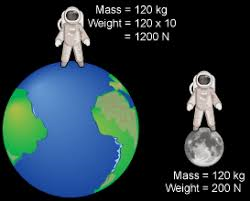* Activity 1.29   :   Reviewing balanced forces and Newtons laws.          ( 10/16/17   Monday )

1.29.a    Balanced force wordsearch.     ( 5 points ).

1.29.b    Newtons laws review.     ( 5 points ).

1.29.c    Tug of war balanced force.    ( 5 points ).

* Activity 1.30   :   Halloween writing.                                                   ( 10/17/17   Tuesday )

1.30.a   Halloween writing to spanish students (intercultural Exchange).   ( 10  points ).

* Activity 1.31   :   Working out forces.                                               ( 10/18/17   Wednesday )

1.31.a   Look the following web up :    Work out the following forces.       ( 5 points ).
( Check the example done ).

1.31.b   Now work out the forces at the following data table.        ( 10  points ).

Use your chromebook to creat a table in which you work out
the value of the forces, acceleratios or masses asked.

1.31.c   A truck has a mass of 15,000 kg. The driving force created  by the engine
is 21,000 N. Calculate the acceleration caused by this force.   ( 5 points ).

( You have the solutions there to check).

* Activity 1.32   :   Gravity and weight.                                                    ( 10/19/17   Thursday )

*   These are the questions to answer  looking up the following links :

1.32.a   GRAVITY, MASS AND WEIGHT.

1.32.b   MASS AND WEIGHT.

1.32.c   GRAVITY.

Check your weight on other planets.

Here you can work out mass, gravity or weight.

When you finish answering all the questions.           ( 20 points ).

* Activity 1.33   :   Gravity and weight test.                                                 ( 10/20/17   Friday )

GRAVITY , MASS AND WEIGHT TEST. ( 10  points ).

Newtons laws quizzes.

## 7th GRADE SCIENCE : Article 20 : Ending Forces lesson 1. Final and review.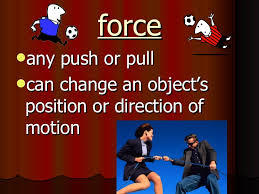* Activity 1.34   :   Force lesson 1.     Reviews.                                       ( 10/23/17   Monday )

1.34.a   Pics of earth, gravity and weight to make the poster.         ( 10 points ).

1.34.b   On the poster you must write a weight and gravitys description.   ( 5 points ).

1.34.c   Fun way to review forces.              ( 5 points ).

Copy this outline on a sheet in order to review some of the forces studied.

* Activity 1.35   :   Listing the main forces studied at the LESSON 1.         ( 10/24/17   Tuesday )

FINAL REVIEW  LESSON 1 :
FORCES AND MOTION.

- These are the main concepts you have studied in this unit :

( What a force is, forces effects, vectors, kind of forces, tension force, spring force, air resistance force, nuclear force, gravitational force, friction force, normal force, electrical force, magnetic force, applied force, contact forces, non-contact forces, weight force, gravity force, electromagnet, earths inner forces, tectonic plates, elastic force, dynamometer, escalar and vectorial magnitudes, application point, speed, velocity, direction, opposite direction, units, newtons, Isaac Newton, Robert Hooke, acceleration, Newtons laws, Hookes law, motion, inertia, F=K.X , F= m.a , balance force, stiffen structures, calculations, net force, reaction force, etc ).

1.35.a   Look up the following links and do a list in google docs with the forces studied in this first lesson .  As well as naming them, explain something about 15 of them describing what they are and which effects they can cause.

-  When you finish email it to me.

( 20  points ).

LINK 1   ( Kind of forces ).

LINK 2  ( Weight , mass , gravity ).

LINK 3  ( Tectonic plates ).

LINK 4  ( Magnitude and vectors , direction ).

LINK 6    ( Elastic force , Hookes law ).

LINK 7  ( Newtons laws, acceleration , motion , inertia ).

LINK 8  ( Net force game ).

LINK 9  ( Net force ).

LINK 10  ( Stiffening structures ).

LINK 11  ( Earth's layers ).

## 7th GRADE SCIENCE : Article 21 : LESSON 2 ENERGY.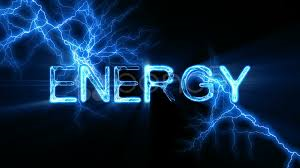LESSON 2

ENERGY.

* Activity 2.1   :   Energy  lesson 2.                                                        ( 10/25/17   Wednesday )

2.1.b     Answer the following questions about Energy at the given paper.  ( 10 points ).

2.1.c       Why is James Prescott Joule so important related to Energy?.

* Activity 2.2   :   Energy  lessons 2 parts.                                              ( 10/26/17   Thursday )

2.2.a       Copy this important issues about Energy in your paper in order to
organize the different aspects you are going to learn about ENERGY.
( 10 points ).

2.2.b        Fill out the blanks with the words given in order to connect forces
with energy.                                                                                           ( 10 points ).

2.2.c        Write in a paper a table with the different forms energy can come.

( 5 points ).

* Activity 2.3   :   Science fair.                                                                   ( 10/27/17   Friday )

2.3.a       Look up this web, do the survey, read the information, choose
the subject of your work for the science fair, send it all to me by

"EVERYBODY MUST DO A SCIENCE PROJECT AT HOME".

YOUR PROJECT MUST BE DONE AT HOME AND WHEN YOU
FINISH IT YOU MUST MAKE A POWERPOINT SHOWING HOW
YOU DID IT, THE SCIENTIFIC METHOD USED, MATERIALS USED,
ETC.
DEADLINE :  FIRST WEEK OF DECEMBER.

SCIENCE FAIR

.
( 10 points ).

2.3.b                   Scientific method for achieving your science fair Project.

- Make a summary about what it is.
- What are the main steps in scientific method.
- What these steps consist of.
- What a hypothesis is.
- What a theory is.
- How a hypothesis or theory becomes a law.
- How to apply all this to your work.

Scientific method example 3.

Scientific method example 4.

Scientific method example 5.

( 10 points ).

## Intercultural exchanges 2017-2018. KENTUCKY - BARCELONA (SPAIN) ARTICLE 1.

Publicado: 24/10/2017 05:34 por easyfisquim en Intercultural exchanges 2017-2018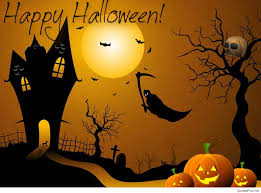INTERCULTURAL EXCHANGE.

KENTUCKY (USA) - BARCELONA (SPAIN)

28 October - 2017.

HALLOWEEN WRITINGS.

( Celebrating Halloween from different countries ).

3. Kentucky  ( 7th grade students writings )  :

## 7th GRADE SCIENCE : Article 22 : Scientific method.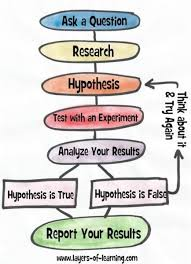* Activity 2.4   :   Scientific method.                                                            ( 10/30/17   Monday )

SCIENTIFIC METHOD.

2.4.a                      Copy the scientific method steps from the classrooms whiteboard.
( 5 points ).

following questions at the paper given to you.

Page with questions to be answered.      ( 10 points ).

2.4.c                   Scientific method for achieving your science fair Project.

- Make a summary about what it is.
- What are the main steps in scientific method.
- What these steps consist of.
- What a hypothesis is.
- What a theory is.
- How a hypothesis or theory becomes a law.
- How to apply all this to your work.

Scientific method example 3.

Scientific method example 4.

Scientific method example 5.

( 10 points ).

- These PAPERS can help you to fill out the different steps you need to make the
scientific method about your Project.  Look them up and bring them home.

* Activity 2.5   :   Example for the scientific method.                                   ( 10/31/17   Tuesday )

2.5.a       Hummingbirds story and the scientific method.                   ( 15 points ).

2.5.b       Wordsearch scientific method.                                                ( 5 points ).

* Activity 2.6   :   Hummingbirds Poster.                                                 ( 11/1/17   Wednesday )

2.6.a    Hummingbirds poster  ( Applying scientific method )        ( 15 points ).

non renewable energies.                                                        ( 10  points ).

* Activity 2.7   :   Renewable and non-renewable energies.                  ( 11/2/17   Thursday )

RENEWABLE AND NON-RENEWABLE ENERGIES.

2.7.a  Open a google docs and make a table listing RENEWABLE and
NON-RENEWABLE energies.

2.7.b  Explain in your google docs the main difference between the renewable and
non-renewable kind of energies.

2.7.c   Make other google docs and explain what consists of renewables energies
like biomass, wind energy, hydro water energy and geotermal energy.

2.7.d   How is biomass converted into energy?.  Put an image to explain the process.

2.7.e   Why is petroleum a non renewable kind of energy?.

* Activity 2.8   :   Organising renewable energy sources.                         ( 11/3/17   Friday )

2.8.a  Complete the papers given to you today about RENEWABLE energy
sources and do the different exercises you have on them.

Papers with exercises given to you today.